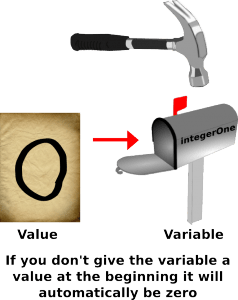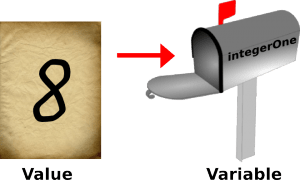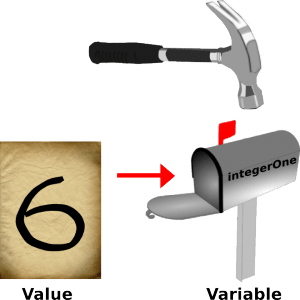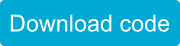# Integer Numbers

SparkiDuino
Easy

### Lessons You Should Know

There are two basic types of numbers you will need to worry about when writing code for Sparki or Hexy: integers and floating point numbers (also know as numbers with decimal points) Integers are numbers without a decimal point. An integer in SparkiDuino can be a positive or negative number anywhere from -2,147,483,648 to +2,147,483,647. Most of the time when you need to store a number, you will use an integer. Here’s what we’ll be going over in this lesson about integers:

#### Declaring an Integer Variable

Before you can store a number you need to create a container to hold that number. If we think of variables like mailboxes to hold information and we think of the values stored inside the variables as letters then declaring a variable is kind of like putting a mailbox into the ground. Before you can get any information out of the mailbox you first have to put it somewhere so that it can receive letters! This is called declaring a variable. You can declare an integer using the word “int” followed by the name you want to give to the variable.This will create a variable named “integerOne.” The type of this variable will be an integer. Since you didn’t give the variable a value when you first created it the value of the variable is automatically zero. Some programming languages will give empty variables a value called “null” but SparkiDuino is intended for beginners so it assigns new variables the value of zero.Assigning Values (Putting Information in the Variables)

You can then change the variable by assigning a value to the variable. This is done simple by writing the variable name followed by an equals sign, followed by the value you want to put in the variable. Then you end your command for assigning a variable with the all important semicolon. Everything else is pretty self explanatory but the semicolon is there so the computer knows that it has reached the end of a line of instructions that should now be executed. You can think of the semicolon as a green light that tells the computer it has all the parts of a command and it can start moving ones and zeros around. Here’s what it looks like when we assign the value of eight to the integer variable “integerOne.”You can think of an integer variable like a mailbox with a letter in it. You can write any number on the paper inside the letter and the robot will look inside the letter anytime it sees the variable name that is on the mailbox. Then the robot will just insert whatever number is on the letter into the code it is running in place of the variable. You can also declare an integer and assign a number to the variable in the same line of code. Here’s what it looks like to declare an integer variable named “integerTwo ” with the initial value of six:These ways to declare, assign and declare assigned are the same for integers, floating points and characters. The difference is that with integer variables you use the word int, but with a float you use the word float and with a character you use the word char at the beginning of each declaration.

#### Doing Math with Integers

Integers are good when you don’t need accuracy. They are great for counting, adding, subtracting and multiplying. Let’s do some basic math using integer variables. We’ll create two variables, one named “foo” and another named “blerp.”You will see the result printed out on Sparki’s LCD screen:This simple math looks correct, until the last line printed out. We know that 10/4 = 2.5, and not 2 like it says on the screen. What happened? Integers do not have decimal points! This makes them bad when you do not know if a value you want to store in them will be a whole number or not. This also makes them bad when you need to divide things, because the left over part will be removed. That’s right, Sparki just throws the decimal point away as well as any other numbers after the decimal point. It won’t even round up if the number is over five!  You will need a floating point number any time you suspect there might possibly be decimals involved.

#### Shorthand Math with Integers

Programmers are lazy so there are ways to do addition and subtraction operations that look a little different because they take less time to type but do the same thing as math commands that take longer to type. Below are examples of shorthand addition followed by the same code, only written out longhand.  For adding to a variable programmers will often use this command- For subtracting from a variable programmers will often use this command- In fact programmers are so lazy there are even extra special shorthand commands for adding or subtracting one with a variable! Here’s what it looks like when programmers use shorthand to add one to an integer variable- Here’s what it looks like when programmers use shorthand to subtract one from a variable-

#### Creating a Random Integer

(Feel free to skip this if you’re new to coding and a little overwhelmed.) Before we leave integers let’s talk about a neat little command that allows you to ask Sparki to make a random integer for you. (It’s not really random, but it’s pretty close.) This is useful for making Sparki act a little less like a robot or for choosing variables values that you don’t really care about too much. The command you will use is random( ). We’ll need to do a couple more advanced things to make this command work, first we need to create a variable to hold this random value and then we need to use the random( ) command with one or two numbers inside the parentheses. These numbers will control the highest and lowest possible number that our random command can create. Below is an example of the two ways you can use this command for creating a random number (you’ll need to include the sparki library and add a setup function to make this code work on Sparki): This is a slightly advanced concept, so don’t feel like you have to really understand it or use it yet, it’s just good for you to see more examples of code that are specific to integer variables so that you’ll feel more comfortable the next time you come across them.

or

## Next Step:

Now that you feel a little more comfortable with integer variables let’s move on to decimal points with floating point numbers….